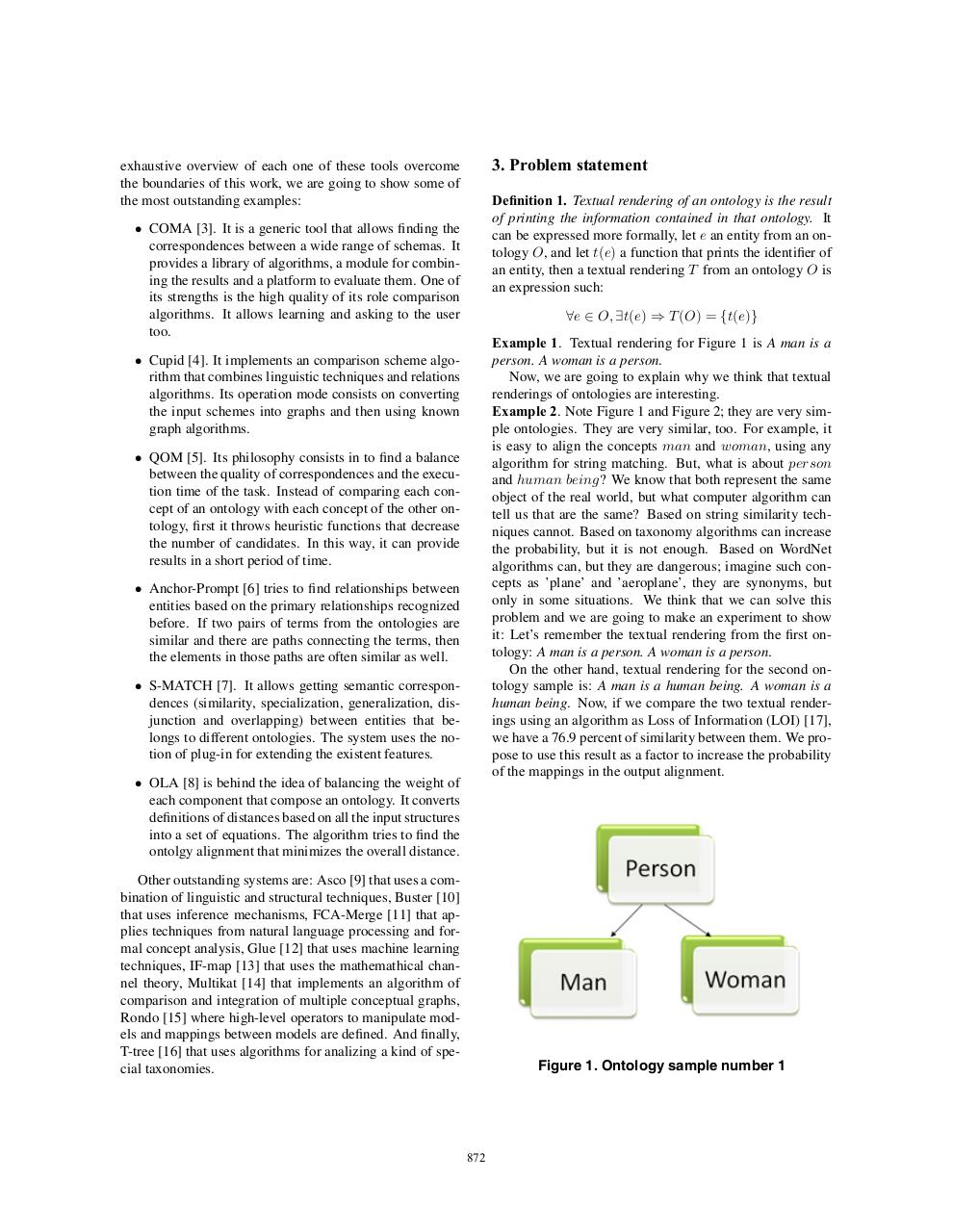# Textual Renderings Ontologies.pdfPage 1 2 3 4 5 6

#### Text preview

3. Problem statement

exhaustive overview of each one of these tools overcome
the boundaries of this work, we are going to show some of
the most outstanding examples:

Definition 1. Textual rendering of an ontology is the result
of printing the information contained in that ontology. It
can be expressed more formally, let e an entity from an ontology O, and let t(e) a function that prints the identifier of
an entity, then a textual rendering T from an ontology O is
an expression such:

• COMA . It is a generic tool that allows finding the
correspondences between a wide range of schemas. It
provides a library of algorithms, a module for combining the results and a platform to evaluate them. One of
its strengths is the high quality of its role comparison
algorithms. It allows learning and asking to the user
too.

∀e ∈ O, ∃t(e) ⇒ T (O) = {t(e)}
Example 1. Textual rendering for Figure 1 is A man is a
person. A woman is a person.
Now, we are going to explain why we think that textual
renderings of ontologies are interesting.
Example 2. Note Figure 1 and Figure 2; they are very simple ontologies. They are very similar, too. For example, it
is easy to align the concepts man and woman, using any
algorithm for string matching. But, what is about person
and human being? We know that both represent the same
object of the real world, but what computer algorithm can
tell us that are the same? Based on string similarity techniques cannot. Based on taxonomy algorithms can increase
the probability, but it is not enough. Based on WordNet
algorithms can, but they are dangerous; imagine such concepts as ’plane’ and ’aeroplane’, they are synonyms, but
only in some situations. We think that we can solve this
problem and we are going to make an experiment to show
it: Let’s remember the textual rendering from the first ontology: A man is a person. A woman is a person.
On the other hand, textual rendering for the second ontology sample is: A man is a human being. A woman is a
human being. Now, if we compare the two textual renderings using an algorithm as Loss of Information (LOI) ,
we have a 76.9 percent of similarity between them. We propose to use this result as a factor to increase the probability
of the mappings in the output alignment.

• Cupid . It implements an comparison scheme algorithm that combines linguistic techniques and relations
algorithms. Its operation mode consists on converting
the input schemes into graphs and then using known
graph algorithms.
• QOM . Its philosophy consists in to find a balance
between the quality of correspondences and the execution time of the task. Instead of comparing each concept of an ontology with each concept of the other ontology, first it throws heuristic functions that decrease
the number of candidates. In this way, it can provide
results in a short period of time.
• Anchor-Prompt  tries to find relationships between
entities based on the primary relationships recognized
before. If two pairs of terms from the ontologies are
similar and there are paths connecting the terms, then
the elements in those paths are often similar as well.
• S-MATCH . It allows getting semantic correspondences (similarity, specialization, generalization, disjunction and overlapping) between entities that belongs to different ontologies. The system uses the notion of plug-in for extending the existent features.
• OLA  is behind the idea of balancing the weight of
each component that compose an ontology. It converts
definitions of distances based on all the input structures
into a set of equations. The algorithm tries to find the
ontolgy alignment that minimizes the overall distance.
Other outstanding systems are: Asco  that uses a combination of linguistic and structural techniques, Buster 
that uses inference mechanisms, FCA-Merge  that applies techniques from natural language processing and formal concept analysis, Glue  that uses machine learning
techniques, IF-map  that uses the mathemathical channel theory, Multikat  that implements an algorithm of
comparison and integration of multiple conceptual graphs,
Rondo  where high-level operators to manipulate models and mappings between models are defined. And finally,
T-tree  that uses algorithms for analizing a kind of special taxonomies.

Figure 1. Ontology sample number 1

872